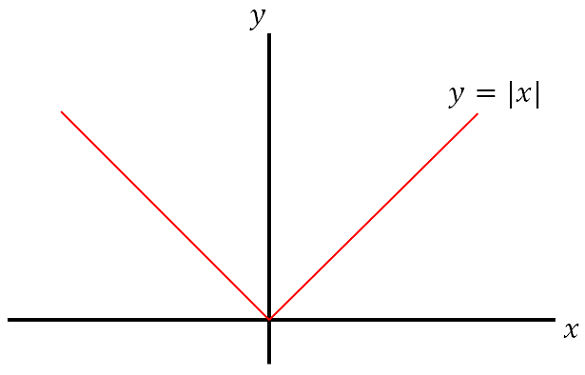# Absolute Value

## Definition

For a real number, the absolute value of $$x$$, denoted $$\lvert x \rvert$$, is defined as

$\left|x \right| = \begin{cases} x, & \text{if } x \geq 0 \\ -x, & \text{if } x < 0. \end{cases}$## Technique

### Evaluate $\left| 3\left( 17-55 \right) \right|$.

\begin{aligned} \left| 3\left( 17-55 \right) \right| &= \left| 3\left( -38 \right) \right| \\ &= \left| -114 \right| \\ &= 114 _\square \end{aligned}

### If $x=\frac{5+\sqrt{11}i}{2}$ and $y=\frac{5 -\sqrt{11}i}{2}$, what is the value of $\left| x + 3y \right|^2$?

\begin{aligned} \left| x + 3y \right|^2 &= \left| \frac{5+\sqrt{11}i}{2} + 3\left( \frac{5 -\sqrt{11}i}{2} \right) \right|^2 \\ &= \left| \frac{5+\sqrt{11}i + 15 -3\sqrt{11}i}{2} \right|^2 \\ &= \left| \frac{20-2\sqrt{11}i}{2} \right|^2 \\ &= \left| 10 - \sqrt{11}i \right|^2 \\ &= \left( \sqrt{10^2 + \sqrt{11}^2} \right)^2 \\ &= \sqrt{111}^2 \\ &= 111 _\square \end{aligned}

### What is the absolute value of $2 - 10a$?

The answer depends on the value of $a$. From the definition above, we have

$\left|2-10a \right| = \begin{cases} 2-10a, & \text{if } 2-10a \geq 0 \\ -(2-10a), & \text{if } 2-10a < 0 \end{cases}$

Solving the inequality on the right, we obtain

\begin{aligned} 2-10a &\geq 0 \\ 2 & \geq 10a \\ \frac{1}{5} &\geq a. \end{aligned}

It follows that $2-10a \geq 0 \Longleftrightarrow a \leq \frac{1}{5}.$

Therefore,

$\left|2-10a \right| = \begin{cases} 2-10a, & \text{if } a \leq \frac{1}{5} \\ 10a - 2, & \text{if } a > \frac{1}{5} \end{cases} _\square$Note by Arron Kau
7 years, 4 months ago

This discussion board is a place to discuss our Daily Challenges and the math and science related to those challenges. Explanations are more than just a solution — they should explain the steps and thinking strategies that you used to obtain the solution. Comments should further the discussion of math and science.

When posting on Brilliant:

• Use the emojis to react to an explanation, whether you're congratulating a job well done , or just really confused .
• Ask specific questions about the challenge or the steps in somebody's explanation. Well-posed questions can add a lot to the discussion, but posting "I don't understand!" doesn't help anyone.
• Try to contribute something new to the discussion, whether it is an extension, generalization or other idea related to the challenge.

MarkdownAppears as
*italics* or _italics_ italics
**bold** or __bold__ bold
- bulleted- list
• bulleted
• list
1. numbered2. list
1. numbered
2. list
Note: you must add a full line of space before and after lists for them to show up correctly
paragraph 1paragraph 2

paragraph 1

paragraph 2

[example link](https://brilliant.org)example link
> This is a quote
This is a quote
    # I indented these lines
# 4 spaces, and now they show
# up as a code block.

print "hello world"
# I indented these lines
# 4 spaces, and now they show
# up as a code block.

print "hello world"
MathAppears as
Remember to wrap math in $$ ... $$ or $ ... $ to ensure proper formatting.
2 \times 3 $2 \times 3$
2^{34} $2^{34}$
a_{i-1} $a_{i-1}$
\frac{2}{3} $\frac{2}{3}$
\sqrt{2} $\sqrt{2}$
\sum_{i=1}^3 $\sum_{i=1}^3$
\sin \theta $\sin \theta$
\boxed{123} $\boxed{123}$

Sort by:

thank you very much :-)

- 7 years, 3 months ago

I like this format of note, I think I'll Start Share So !

Explanation More Exercises Solved !

- 7 years, 4 months ago

I didn't quite understand the problem having $i$. At one stage the $i$ disappears. Can you please explain?

- 7 years, 4 months ago

It is because the absolute value of a complex number ($a + bi$) is defined as $\sqrt{a^2 + b^2}$ , i.e., $|a + bi|$ = $\sqrt{a^2 + b^2}$. In the above question, $|10 - \sqrt11|$ = $\sqrt{10^2 + \sqrt{11}^2}$

- 7 years, 4 months ago

Didn't know that, thanks!!

- 7 years, 4 months ago

I am not sure but as far as my mind think I can say this is because when the sign of absolute value was removed.Then sign was changed to positive & i(iota) has nothing to do when there is is postive root.So,It would have been removed...

- 7 years, 4 months ago

$i$ is defined as $\sqrt{-1}$ i.e. imaginary unit. So, those were complex numbers (real number + imaginary numbers).

- 7 years, 4 months ago

Excellent...

- 7 years, 4 months ago

In ending step ..note that to take modulus of imag eniry number is different than simple modulas

- 6 years, 9 months ago